This document contains R versions of the boxed examples from Chapter 12 of the “Analysis and Interpretation of Freshwater Fisheries Data” book. Some sections build on descriptions from previous sections, so each section may not stand completely on its own. More thorough discussions of the following items are available in linked vignettes:

The following additional packages are required to complete all of the examples (with the required functions noted as a comment and also noted in the specific examples below).

> library(FSA)          # Subset, Summarize, residPlot
> library(car)          # Anova, recode
> library(plyr)         # ddply

In addition, external tab-delimited text files are used to hold the data required for each example. These data are loaded into R in each example with read.table(). Before using read.table() the working directory of R must be set to where these files are located on your computer. The working directory for all data files on my computer is set with

> setwd("C:/aaaWork/Web/fishR/BookVignettes/aiffd2007")

In addition, I prefer to not show significance stars for hypothesis test output, reduce the margins on plots, alter the axis label positions, and reduce the axis tick length. In addition, contrasts are set in such a manner as to force R output to match SAS output for linear model summaries. All of these options are set with

> options(width=90,continue=" ",show.signif.stars=FALSE,
contrasts=c("contr.sum","contr.poly"))
> par(mar=c(3.5,3.5,1,1),mgp=c(2.1,0.4,0),tcl=-0.2)

## 12.1 Scope for Growth

THERE IS NO CODE TO CONVERT FOR THIS BOX

## 12.2 Sample Analysis of Striped Bass Data

Presented here is a step-by-step account of an approach to analyzing common energetic data such as $$C_{max}$$ or metabolism. In the example provided here, data are from maximum consumption experiments for Striped Bass (Morone saxatilis) conducted by Hartman (1993). The data set (available in the Chapter 12 compact disc (CD) folder) is arranged by the variables wet weight, temperature, and ration.

### 12.2.1 Preparing Data

The Box12_2.txt data file is read and the structure of the data frame is observed below.

> d2 <- read.table("data/Box12_2.txt",header=TRUE)
> str(d2)
'data.frame':   170 obs. of  3 variables:
$wt : num 56.9 41.5 52.4 27.9 29.3 ...$ temp: num  2.04 2.16 2.21 2.25 2.26 2.35 2.38 2.43 2.46 2.46 ...
$Cmax: num 0 0.00175 0.0095 0 0.00768 ... In addition, I created a new variable, called tempA, that contains the temperature groupings used to construct Figure A in the box. The temperature groupings were identified by examining the Excel file provided on the companion CD. > d2$tempA <- NA
> d2$tempA[d2$temp>6 & d2$temp<9] <- 6.9 > d2$tempA[d2$temp>21 & d2$temp<24] <- 22.4
> d2$tempA[d2$temp>28 & d2$temp<31] <- 29.6 > d2$tempA <- factor(d2$tempA) > str(d2) 'data.frame': 170 obs. of 4 variables:$ wt   : num  56.9 41.5 52.4 27.9 29.3 ...
$temp : num 2.04 2.16 2.21 2.25 2.26 2.35 2.38 2.43 2.46 2.46 ...$ Cmax : num  0 0.00175 0.0095 0 0.00768 ...
$tempA: Factor w/ 3 levels "6.9","22.4","29.6": NA NA NA NA NA NA NA NA NA N.. Another new variable, called wtA, was created to contain the size groupings used to construct Figure B in the box. The cutoff values were as stated in the caption for Figure B. > d2$wtA <- NA
> d2$wtA[d2$wt<77] <- 38
> d2$wtA[d2$wt>130 & d2$wt<730] <- 403 > d2$wtA[d2$wt>800] <- 1567 > d2$wtA <- factor(d2$wtA) > str(d2) 'data.frame': 170 obs. of 5 variables:$ wt   : num  56.9 41.5 52.4 27.9 29.3 ...
$temp : num 2.04 2.16 2.21 2.25 2.26 2.35 2.38 2.43 2.46 2.46 ...$ Cmax : num  0 0.00175 0.0095 0 0.00768 ...
$tempA: Factor w/ 3 levels "6.9","22.4","29.6": NA NA NA NA NA NA NA NA NA N..$ wtA  : Factor w/ 3 levels "38","403","1567": 1 1 1 1 1 1 1 1 1 1 ...

### 12.2.2 Plots

The authors fit a power function to the maximum consumption versus weight variables for the 22.4 and 29.6 degrees grouping and a linear function to the 6.9 degrees group. The power functions are fit as linear models on the log-log data. The code below first transforms the maximum consumption and weight variables to the common log scale. The linear model for the 6.9 group is then fit with lm() using a formula of the form response~explanatory, submitting the data file containing the variables in the data= argument, and then using the subset= argument to isolate the 6.9 group. The model coefficients are extracted from the saved lm object with coef(). Linear models are fit to the log-log data for the other two groups.

> d2$logwt <- log10(d2$wt)
> d2$logCmax <- log10(d2$Cmax)
> lm.low <- lm(Cmax~wt,data=d2,subset=tempA=="6.9")
> coef(lm.low)
 (Intercept)           wt
1.626563e-02 1.809897e-06 
> lm.med <- lm(logCmax~logwt,data=d2,subset=tempA=="22.4")
> coef(lm.med)
(Intercept)       logwt
-0.5995662  -0.2477909 
> lm.hi  <- lm(logCmax~logwt,data=d2,subset=tempA=="29.6")
> coef(lm.hi)
(Intercept)       logwt
-1.08058424 -0.04378962 

Figure A is constructed in parts by first making a raw schematic plot (note that type="n" will result in no points or lines being plotted), then adding points for each grouping, and then adding the curves derived from the coefficients for each grouping.

> # schematic plot
> plot(Cmax~wt,data=d2,type="n",xlab="Wet weight (g)",ylab="Cmax(g/g/d)")
> # add points specific to each group
> points(Cmax~wt,data=d2,subset=tempA=="6.9",pch=19,col="red")
> points(Cmax~wt,data=d2,subset=tempA=="22.4",pch=19,col="blue")
> points(Cmax~wt,data=d2,subset=tempA=="29.6",pch=19,col="black")
> # add the fitted curve specific to each group
> curve(coef(lm.low)+coef(lm.low)*x,min(d2$wt[d2$tempA=="6.9"],na.rm=TRUE),
max(d2$wt[d2$tempA=="6.9"],na.rm=TRUE),add=TRUE,col="red",lwd=2)
> curve(10^(coef(lm.med))*x^coef(lm.med),min(d2$wt[d2$tempA=="22.4"],na.rm=TRUE),
max(d2$wt[d2$tempA=="22.4"],na.rm=TRUE),add=TRUE,col="blue",lwd=2)
> curve(10^(coef(lm.hi))*x^coef(lm.hi),min(d2$wt[d2$tempA=="29.6"],na.rm=TRUE),
max(d2$wt[d2$tempA=="29.6"],na.rm=TRUE),add=TRUE,col="black",lwd=2)
> legend("topright",legend=c("6.9C","22.4C","29.6C"),pch=19,col=c("red","blue","black"),
lty=1,lwd=2)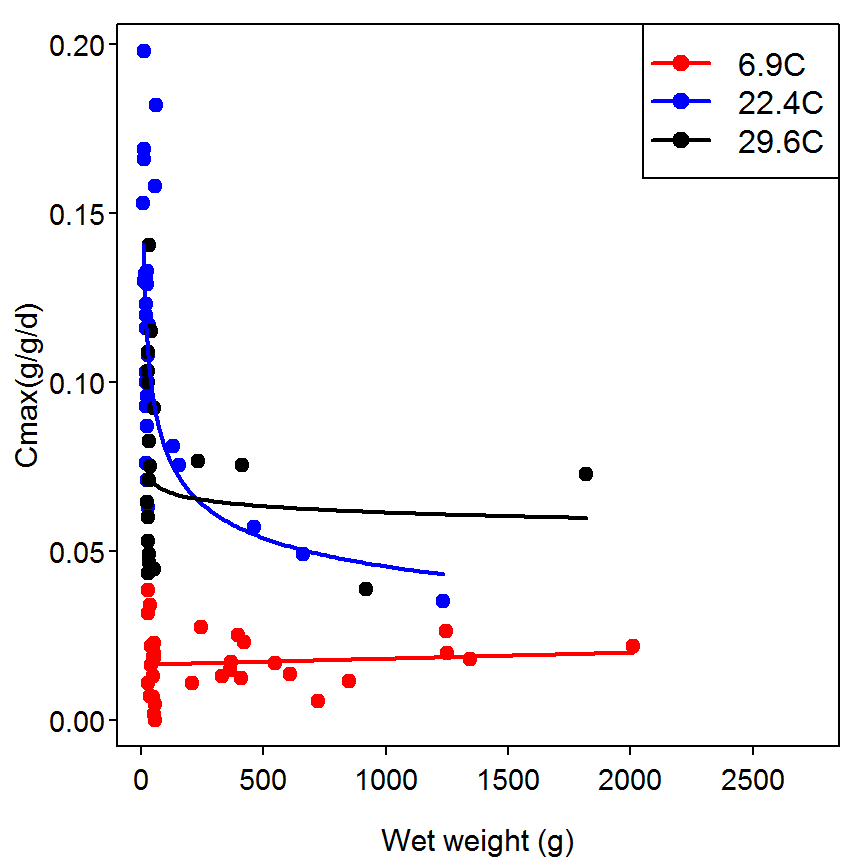The polynomial regressions for the relationship between maximum consumption and temperature shown in Figure B are also fit with lm() using a formula that represents the quadratic function. Note in the formula that I() must be wrapped around the quadratic term so that R will know to actually square that term (i.e., the \^2 notation has a special meaning in the model formula if not contained within I()). Figure B is then constructed in parts as described for Figure A.

> lm.sm <- lm(Cmax~temp+I(temp^2),data=d2,subset=wtA=="38")
> coef(lm.sm)
  (Intercept)          temp     I(temp^2)
-0.0375854340  0.0119047823 -0.0002664605 
> lm.int <- lm(Cmax~temp+I(temp^2),data=d2,subset=wtA=="403")
> coef(lm.int)
  (Intercept)          temp     I(temp^2)
-0.0339716353  0.0083666745 -0.0001672247 
> lm.lrg  <- lm(Cmax~temp+I(temp^2),data=d2,subset=wtA=="1567")
> coef(lm.lrg)
  (Intercept)          temp     I(temp^2)
-4.664772e-03  4.204417e-03 -8.317571e-05 
> # The plot
> plot(Cmax~temp,data=d2,type="n",xlab="Temperature (C)",ylab="Cmax(g/g/d)")
> points(Cmax~temp,data=d2,subset=wtA=="38",pch=19,col="red")
> points(Cmax~temp,data=d2,subset=wtA=="403",pch=19,col="blue")
> points(Cmax~temp,data=d2,subset=wtA=="1567",pch=19,col="black")
> curve(coef(lm.sm)+coef(lm.sm)*x+coef(lm.sm)*x^2,min(d2$temp,na.rm=TRUE), max(d2$temp,na.rm=TRUE),add=TRUE,col="red",lwd=2)
> curve(coef(lm.int)+coef(lm.int)*x+coef(lm.int)*x^2,min(d2$temp,na.rm=TRUE), max(d2$temp,na.rm=TRUE),add=TRUE,col="blue",lwd=2)
> curve(coef(lm.lrg)+coef(lm.lrg)*x+coef(lm.lrg)*x^2,min(d2$temp,na.rm=TRUE), max(d2$temp,na.rm=TRUE),add=TRUE,col="black",lwd=2)
> legend("topleft",legend=c("38 g","403 g","1567 g"),pch=19,col=c("red","blue","black"),
lty=1,lwd=2)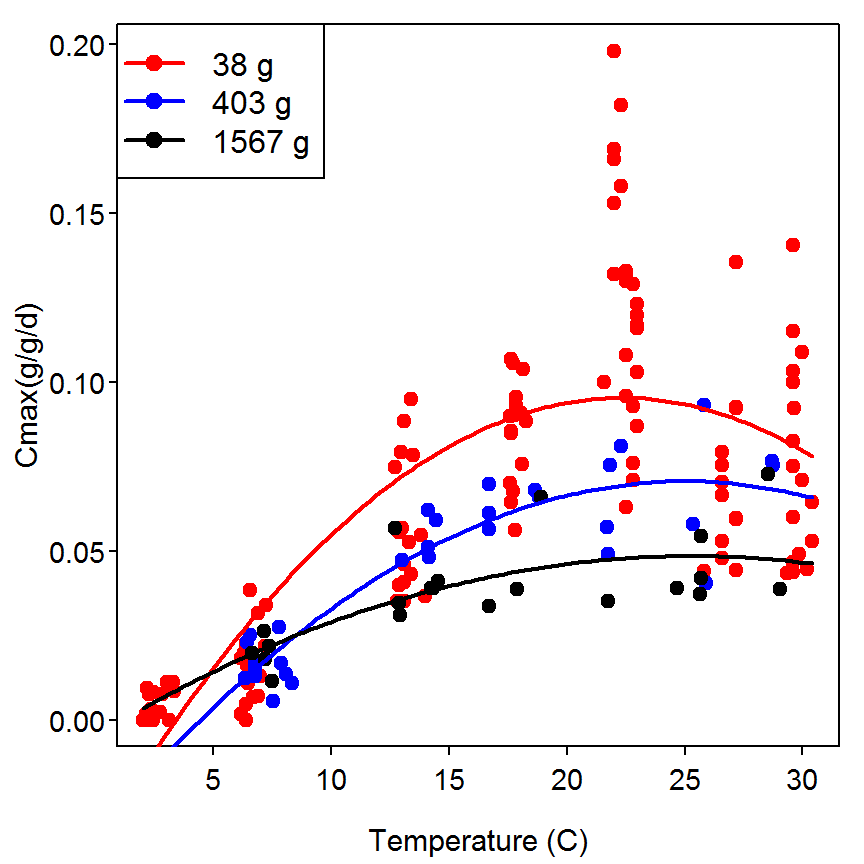### 12.2.3 Test for Significant Interaction Terms

The authors then begin to test for a significant interaction effect of weight and temperature on maximum consumption. Before doing this analysis the authors removed all observations where maximum consumption was equal to zero. This filtering is accomplished with Subset(), from the FSA package. This function requires the orginal data frame as the first argument and the conditioning statement as the second argument.

> d2a <- Subset(d2,Cmax>0)

The linear model is then fit with lm() using a formula of the form response~explanatory1*explanatory2. This formula is a short-hand method to tell R to fit the two main effects and the interaction effect of the two explanatory variables on the right-hand-side of the formula. The type-I ANOVA table is extracted by submitting the saved lm object to anova() and the Type-III ANOVA table is extracted by submitting the saved lm object to Anova() with the type="III" argument. As the authors’ noted, the interation term is not siginificant if a 5% significance level is used.

> lm1 <- lm(logCmax~logwt*temp,data=d2a)
> anova(lm1)
Analysis of Variance Table

Response: logCmax
Df  Sum Sq Mean Sq  F value    Pr(>F)
logwt        1  1.4688  1.4688  18.8713 2.475e-05
temp         1 14.5110 14.5110 186.4424 < 2.2e-16
logwt:temp   1  0.2311  0.2311   2.9698   0.08676
Residuals  160 12.4530  0.0778                   
> Anova(lm1,type="III")
Anova Table (Type III tests)

Response: logCmax
Sum Sq  Df  F value    Pr(>F)
(Intercept) 10.7009   1 137.4886 < 2.2e-16
logwt        0.0605   1   0.7774   0.37926
temp         2.4184   1  31.0718 1.034e-07
logwt:temp   0.2311   1   2.9698   0.08676
Residuals   12.4530 160                   

### 12.2.4 Developing a Statistical Model

The authors then developed a multiple linear regression model to describe maximum consumption based on the logarithm of weight and linear and quadratic temperature terms. This model is again fit with lm(), the ANOVA table is extracted as described above, and the model coefficients are extracted from the lm object with summary().

> lm2 <- lm(logCmax~logwt+temp+I(temp^2),data=d2a)
> anova(lm2)
Analysis of Variance Table

Response: logCmax
Df  Sum Sq Mean Sq F value    Pr(>F)
logwt       1  1.4688  1.4688  41.579 1.277e-09
temp        1 14.5110 14.5110 410.785 < 2.2e-16
I(temp^2)   1  7.0321  7.0321 199.069 < 2.2e-16
Residuals 160  5.6520  0.0353                  
> Anova(lm2,type="III")
Anova Table (Type III tests)

Response: logCmax
Sum Sq  Df  F value    Pr(>F)
(Intercept) 38.518   1 1090.394 < 2.2e-16
logwt        0.876   1   24.793 1.638e-06
temp        11.769   1  333.175 < 2.2e-16
I(temp^2)    7.032   1  199.069 < 2.2e-16
Residuals    5.652 160                   
> summary(lm2)

Call:
lm(formula = logCmax ~ logwt + temp + I(temp^2), data = d2a)

Residuals:
Min       1Q   Median       3Q      Max
-0.91139 -0.10603  0.02387  0.11803  0.36638

Coefficients:
Estimate Std. Error t value Pr(>|t|)
(Intercept) -2.4125435  0.0730606 -33.021  < 2e-16
logwt       -0.1194826  0.0239963  -4.979 1.64e-06
temp         0.1450706  0.0079477  18.253  < 2e-16
I(temp^2)   -0.0032962  0.0002336 -14.109  < 2e-16

Residual standard error: 0.1879 on 160 degrees of freedom
Multiple R-squared:  0.8028,    Adjusted R-squared:  0.7991
F-statistic: 217.1 on 3 and 160 DF,  p-value: < 2.2e-16

### 12.2.5 Evaluate Model Fit

The authors’ Figure C shows the residuals on the original maximum consumption scale plotted against temperature. The residuals are found by first creating predicted maximum consumptions by back-transforming fitted log maximum consumptions. The differences between the observed maximum consumption and this predicted maximum consumption are the residuals plotted in Figure C. The predictions and residual computations are below and then Figure C is constructed in parts largely as described for Figure A above.

> d2a$pCmax <- 10^lm2$fitted
> d2a$resids2 <- d2a$Cmax-d2a$pCmax > plot(resids2~temp,data=d2a,type="n",xlab="Temperature",ylab="Residuals") > points(resids2~temp,data=d2a,subset=wtA=="38",col="red",pch=19) > points(resids2~temp,data=d2a,subset=wtA=="403",col="blue",pch=19) > points(resids2~temp,data=d2a,subset=wtA=="1567",col="black",pch=19) > abline(v=c(5,10,15,20,24,28),col="gray90",lty=3) > legend("topleft",legend=c("38 g","403 g","1567 g"),pch=19,col=c("red","blue","black"), lty=1,lwd=2)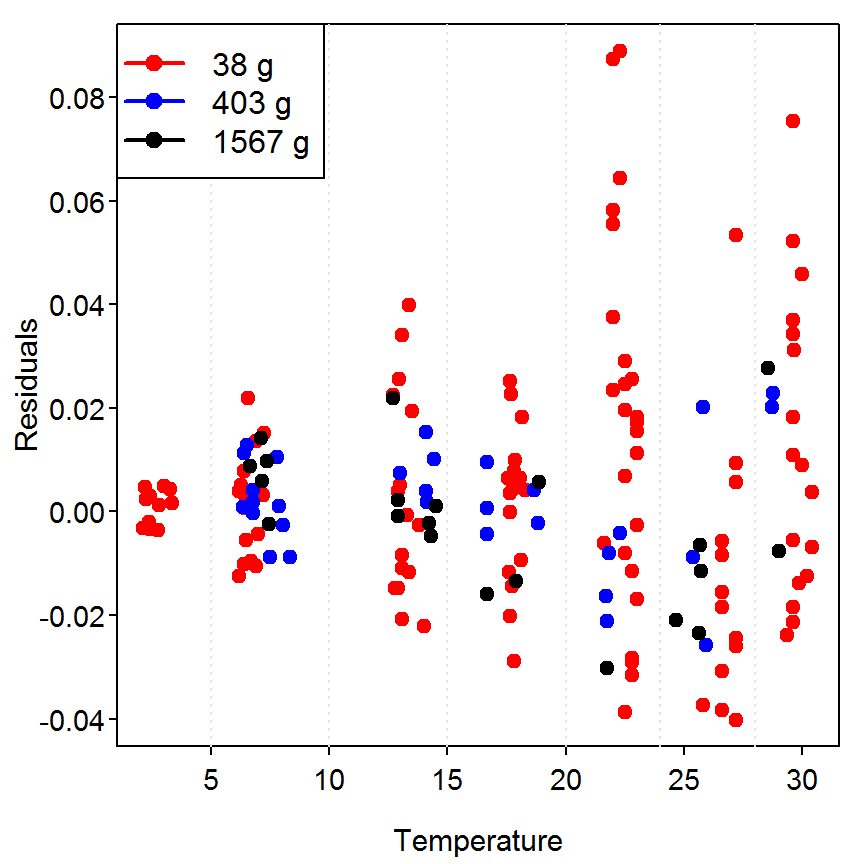The authors’ Figure D was a bit more problematic to construct as they seemed to create “bands” of temperatures for which they summarized the predicted maximum consumptions. They appeared to use different temperature “bands” for different sizes of fish. I chose to create one set of “bands” that are illustrated by the faint gray lines in the plot above. The “bands” were created with recode() (from the car package) rather than the multiple lines depicted for some of the variables above (e.g., tempA). To do this, I created a new variable, called tempB, that contains the groupings for these “bands” and then computed the CV of the predicted maximum consumption for each weight grouping and temperature band using ddply(), from the plyr package. > d2a$tempB <- recode(d2a$temp,"0:5='3'; 6:10='8'; 11:15='13'; 16:20='18'; 21:23.5='22'; 24:28='26'; else='29'") > sumcv <- ddply(d2a,c("wtA","tempB"),function(df) sd(df$pCmax)/mean(df$pCmax)*100) > names(sumcv) <- "CV" > str(sumcv) 'data.frame': 19 obs. of 3 variables:$ wtA  : Factor w/ 3 levels "38","403","1567": 1 1 1 1 1 1 1 2 2 2 ...
$tempB: num 3 8 13 18 22 26 29 8 13 18 ...$ CV   : num  13.03 9.73 4.78 2.05 4.71 ...

Figure D is then constructed in parts as shown below.

> plot(CV~tempB,data=sumcv,type="n",xlab="Temperature (C)",ylab="CV")
> points(CV~tempB,data=sumcv,subset=wtA==38,type="b",pch=19,col="red",lwd=2)
> points(CV~tempB,data=sumcv,subset=wtA==403,type="b",pch=19,col="blue",lwd=2)
> points(CV~tempB,data=sumcv,subset=wtA==1567,type="b",pch=19,col="black",lwd=2)
> legend("topright",legend=c("38 g","403 g","1567 g"),pch=19,col=c("red","blue","black"),
lty=1,lwd=2)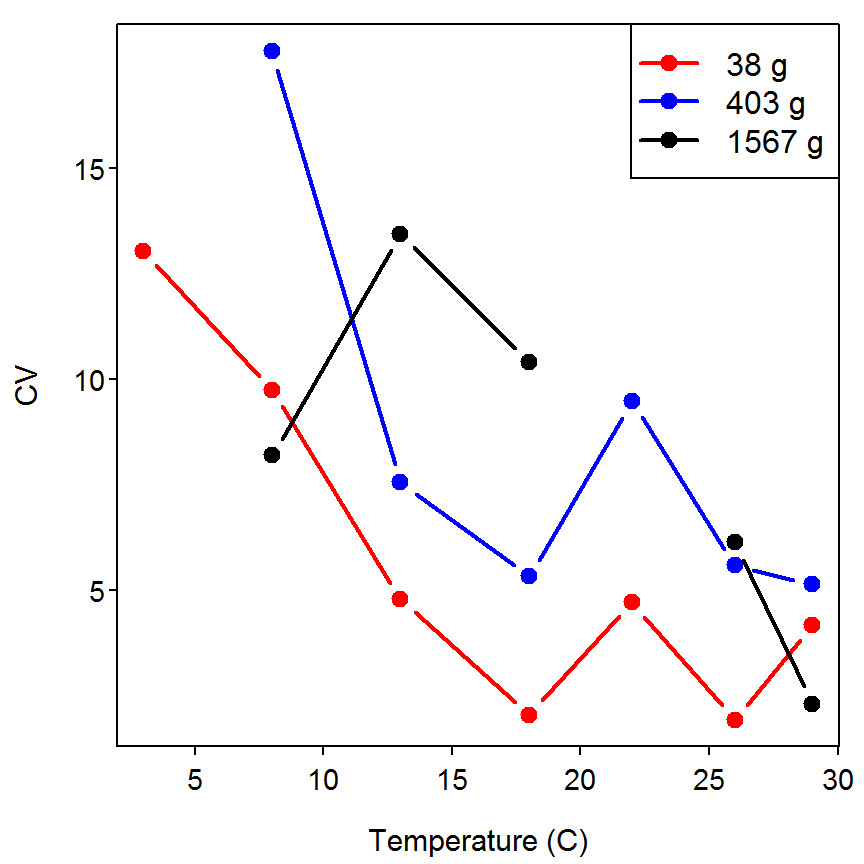This graph does not perfectly match Figure D because I used different “temperature bands.” However, it is functionally the same.

### 12.2.6 Another Residual Plot

In my opinion it is important to look at the residual plot from the actual model fit (i.e., the residuals and fitted values from the log scale). This plot cannot be constructed with residPlot(), from the FSA package. Rather, the residuals and fitted values must be extracted from the lm object and plotted as shown below. There does not appear to be a gross heteroscedasticity or non-linearity in this plot indicating that the assumptions of the linear model were approximately met on the scale to which the model was fit. There does appear to be slightly more variability at the smaller fitted values and a possible outlier.

> plot(lm2$residuals~lm2$fitted,pch=19,xlab="Fitted Values",ylab="Residuals")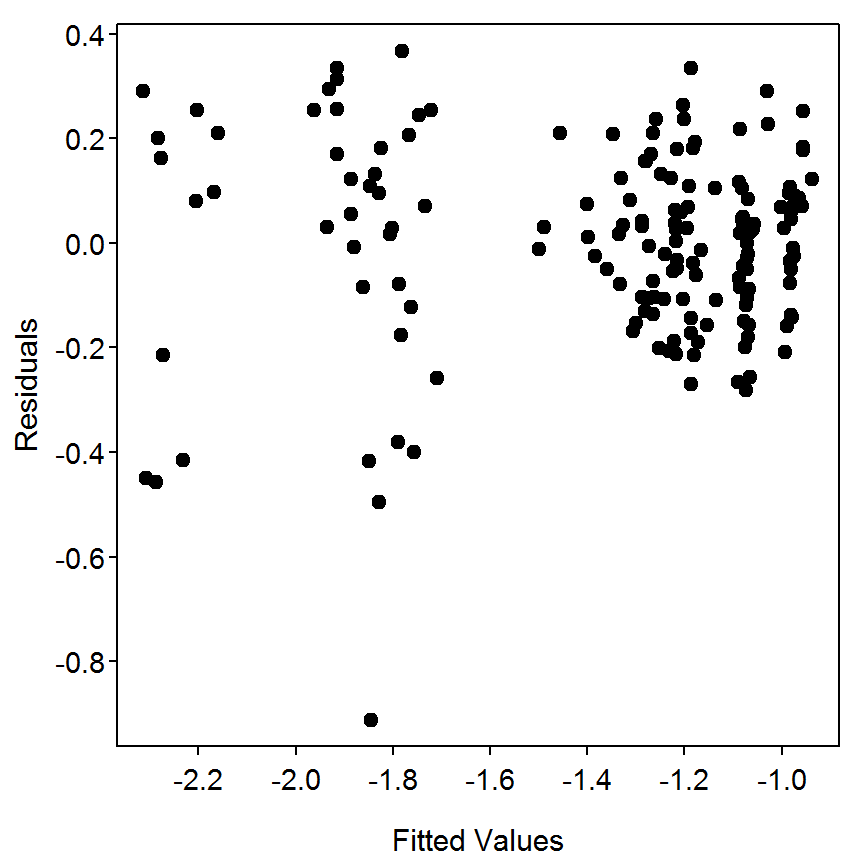Reproducibility Information
Compiled Date: Fri May 15 2015
Compiled Time: 6:10:28 PM
Code Execution Time: 1.22 s

R Version: R version 3.2.0 (2015-04-16)
System: Windows, i386-w64-mingw32/i386 (32-bit)
Base Packages: base, datasets, graphics, grDevices, methods, stats, utils
Required Packages: FSA, car, plyr and their dependencies (dplyr, gdata,
gplots, graphics, Hmisc, knitr, lmtest, MASS, mgcv, multcomp, nnet,
pbkrtest, plotrix, quantreg, Rcpp, relax, sciplot, stats)
Other Packages: car_2.0-25, FSA_0.6.13, knitr_1.10.5, plyr_1.8.2,
zoo_1.7-12
caTools_1.17.1, cluster_2.0.1, codetools_0.2-11, colorspace_1.2-6,
DBI_0.3.1, digest_0.6.8, dplyr_0.4.1, evaluate_0.7, foreign_0.8-63,
formatR_1.2, Formula_1.2-1, gdata_2.16.1, ggplot2_1.0.1, gplots_2.17.0,
grid_3.2.0, gridExtra_0.9.1, gtable_0.1.2, gtools_3.4.2, highr_0.5,
Hmisc_3.16-0, htmltools_0.2.6, KernSmooth_2.23-14, lattice_0.20-31,
latticeExtra_0.6-26, lme4_1.1-7, lmtest_0.9-33, magrittr_1.5,
MASS_7.3-40, Matrix_1.2-0, mgcv_1.8-6, minqa_1.2.4, multcomp_1.4-0,
munsell_0.4.2, mvtnorm_1.0-2, nlme_3.1-120, nloptr_1.0.4, nnet_7.3-9,
parallel_3.2.0, pbkrtest_0.4-2, plotrix_3.5-11, proto_0.3-10,
quantreg_5.11, RColorBrewer_1.1-2, Rcpp_0.11.6, relax_1.3.15,
reshape2_1.4.1, rpart_4.1-9, sandwich_2.3-3, scales_0.2.4,
sciplot_1.1-0, SparseM_1.6, splines_3.2.0, stringi_0.4-1,
stringr_1.0.0, survival_2.38-1, TH.data_1.0-6, tools_3.2.0, yaml_2.1.13

## References

Hartman, K. J. 1993. Striped bass, bluefish, and weakfish in the Chesapeake Bay: Energetics, trophic linkages, and bioenergetics model applications. PhD thesis, University of Maryland, College Park.